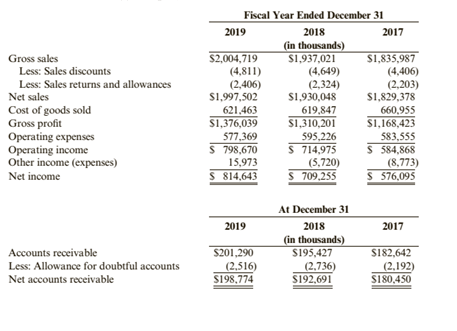Chapter 5, Problem 87APSA### Cornerstones of Financial Accounti...

4th Edition
Jay Rich + 1 other
ISBN: 9781337690881

#### Solutions### Cornerstones of Financial Accounti...

4th Edition
Jay Rich + 1 other
ISBN: 9781337690881
Textbook Problem
14 views

# Ratio AnalysisSelected information from Bigg Company’s financial statements follows:Required:1. Calculate the following ratios for 2018 and 2019: (a) gross profit margin, (b) operating margin, (c) net profit margin, and (d) accounts receivable turnover. ( Note: Round answers to two decimal places.)2. CONCEPTUAL CONNECTION For each of the first three ratios listed above, provide a plausible explanation for any differences that exist. (For example, why is the net profit margin higher or lower than it was the previous year?)3. CONCEPTUAL CONNECTION Explain what each ratio attempts to measure. Make an assessment about Small Company based upon the ratios you have calculated. Are operations improving or worsening?

To determine

(a)

Gross profit margin ratio:

The gross margin ratio is a type of profitability ratio which is used to measure the returns and earning after direct expenses and compute the ratio in respect to the sales of the business.

Operating margin:

The operating margin is the ratio which is used to measure the efficiency of the operations of the business. It is computed by dividing the operating income with the net sales.

Net Profit Margin Ratio:

The net margin ratio is a type of profitability ratio which is used to measure the returns and earning after all expenses i.e. net earnings and compute the ratio in respect to the sales of the business.

Accounts Receivable Turnover Ratio:

The accounts receivable turnover ratio is a ratio which helps in measuring the process of collection of receivables of a business as faster the process, better the liquidity of the business. This ratio shows the policy of the company and its effectiveness against the credit sales of the company.

To calculate:

The gross profit margin, operating margin, net profit margin, and accounts receivables turnover 2018 and 2019.

Explanation

The Big Company has the following financial information:

 Particulars Amounts ($) in 2018 Amounts ($) in    2019 Net Sales 19,30,048 19,97,502 Gross Profit 13,10,201 13,76,039 Operating Income 7,14,975 7,98,670 Net Income 7,09,255 8,14,643 Net Accounts Receivables 1,92,691 1,98,774

The net accounts receivable for the year 2017 is $1,80,450. This is given in the question. The computation of gross profit margin is as follows: Gross Profit Margin (2018)=Gross ProfitNet Sales×100=$13,10,201$19,30,048×100=67.88% Gross Profit Margin (2019)=Gross ProfitNet Sales×100=$13,76,039$19,97,502×100=68.89% The computation of operating margin is as follows: Operating Margin (2018)=Operating IncomeNet Sales×100=$7,14,975$19,30,048×100=37.04% Operating Margin (2019)=Operating IncomeNet Sales×100=$7,98,670\$19,97,502×100=39

To determine

(b)

Gross profit margin ratio:

The gross margin ratio is a type of profitability ratio which is used to measure the returns and earning after direct expenses and compute the ratio in respect to the sales of the business.

Operating margin:

The operating margin is the ratio which is used to measure the efficiency of the operations of the business. It is computed by dividing the operating income with the net sales.

Net Profit Margin Ratio:

The net margin ratio is a type of profitability ratio which is used to measure the returns and earning after all expenses i.e. net earnings and compute the ratio in respect to the sales of the business.

Accounts Receivable Turnover Ratio:

The accounts receivable turnover ratio is a ratio which helps in measuring the process of collection of receivables of a business as faster the process, better the liquidity of the business. This ratio shows the policy of the company and its effectiveness against the credit sales of the company.

The explanation for the differences in the gross profit margin, operating margin, and net profit margin between 2018 and 2019.

To determine

(c)

Gross profit margin ratio:

The gross margin ratio is a type of profitability ratio which is used to measure the returns and earning after direct expenses and compute the ratio in respect to the sales of the business.

Operating margin:

The operating margin is the ratio which is used to measure the efficiency of the operations of the business. It is computed by dividing the operating income with the net sales.

Net Profit Margin Ratio:

The net margin ratio is a type of profitability ratio which is used to measure the returns and earning after all expenses i.e. net earnings and compute the ratio in respect to the sales of the business.

Accounts Receivable Turnover Ratio:

The accounts receivable turnover ratio is a ratio which helps in measuring the process of collection of receivables of a business as faster the process, better the liquidity of the business. This ratio shows the policy of the company and its effectiveness against the credit sales of the company.

The explanation for the differences in the gross profit margin, operating margin, and net profit margin between 2018 and 2019.

### Still sussing out bartleby?

Check out a sample textbook solution.

See a sample solution

#### The Solution to Your Study Problems

Bartleby provides explanations to thousands of textbook problems written by our experts, many with advanced degrees!

Get Started